# Bridging the Gap- Modeling and Methods in Maths(Part 2)

there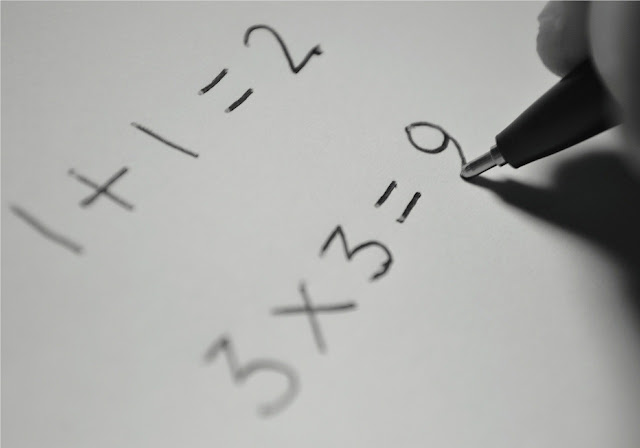Doing maths

Understanding Maths requires one to be able to analyse the question and choose the most appropriate method to solve the question. To be able to decide on which method  to use the child has to be able to  identify the crux of the  problem before he/she is able to slowly show the required workings to solve the problem.

## Using the Model Method

From Primary 1 students are taught to draw models to solve Long Answer Questions. This is the  method that is “promoted” as it is supposedly the easiest method to explain how you can derive the answer. ( And NO you are not allowed to even use Algebra to explain yourself)

Question 1

Sammy and Gerald had an equal number of stamps at first. Sammy then gave away 20 of this stickers to his friend Winston, and Sammy Gerald bought another 12 stamps. In the end, Gerald had thrice as many stickers as Sammy. Find the number of stamps Sammy had at first.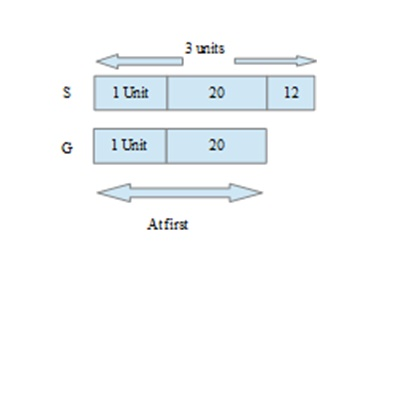Therefore 2 units 20 + 12

1 unit 32 2

1 unit → 16

Number of stamps Gerald had at first = 1 unit + 20

16 + 20

= 36

Gerald had 36 stamps at first.

If you notice the type of model drawn differs from the type of models drawn in lower primary.  It is more complex and you are comparing more then two different items involving multiple steps to solve the question.

## Looking at a Pattern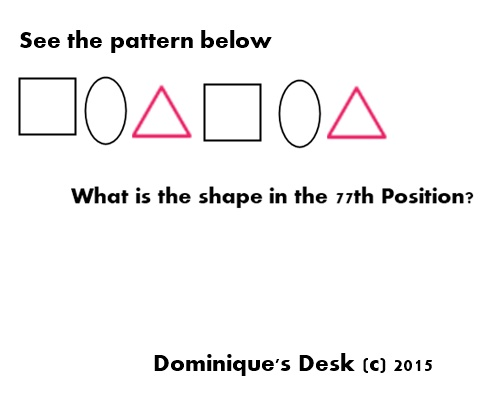Identifying the Pattern

How do you solve this problem?

Firstly you would need to identify the pattern.  Based on the diagram above you would notice that there are 3 different shapes – Square, Oval and Triangle.  The pattern repeats itself after the 3rd shape.

Step 1- Find out the number of “groups of 3” which are possible from 1- 77

77  3 = 25.66 ~ 25 times ( 25 R 2)

25x 3 = 75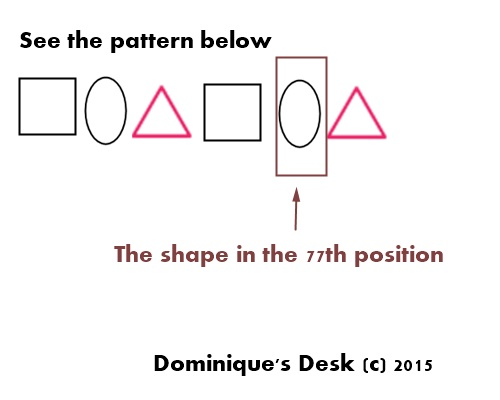It does take a lot of practice to be able to solve a mathematical question easily.   It’s fortunate that Maths is one of Monkey boy’s stronger subject and it doesn’t take him too much effort to understand the concepts which needs to be applied to the various questions.

## How to you reinforce the learning of Maths for your kids?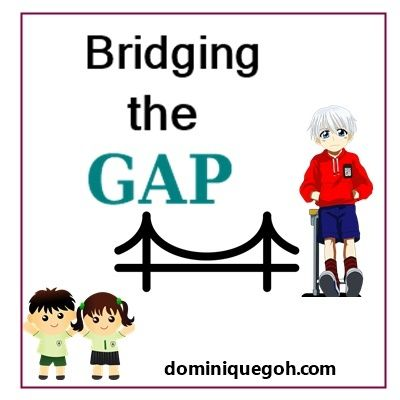Post in this series

9) Bridging the Gap- Upper and Lower Primary Science

10) Bridging the Gap-  Moving from Lower to Upper Primary

11) Bridging the Gap- Moving from Lower to Upper Primary ( Part 2)

# Identifying the Gap between P4 and P5 Maths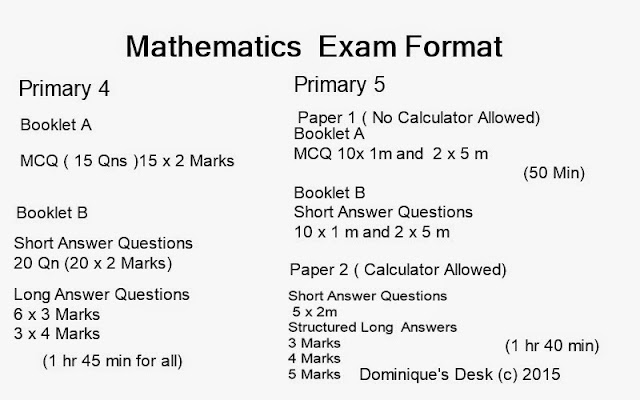The mathematics exam format

Mathematics has always been one of the subjects which the Monkey boy does not have major difficulties in. He has  always been strong in this subject and achieves  between 80-90 for most of his Math’s papers.

However as his move from one level to another there is in an increase in the depth of each topic which he would have to master and maintaining good grades gets harder.

As you can see from the table above there are now two papers that have to be completed for the Maths Exam instead of one.

The time for the exam has been extended from 1hr 45 min to  50 min + 1h 40min. Within the extra 45 min 2 Papers have to be completed instead of just one.

Even though the calculator is allowed to be used for  Paper 2 the questions that are posed are much more complicated and require multiple steps to solve. ( On average 4-6 steps per question)

In  a  Primary 4 Maths exam the student has to complete  44 questions  while in Primary 5 they need to complete 48 questions within an additional 45 minutes.

Even though there are only 4 more questions it can pose much difficult to the student.  These additional questions are within the  Structured Long Questions in Paper 2 which adds up to a   50% weightage of the whole paper.

This certainly discounts the fact that even though the  calculator is allowed for use in Paper 2 it doesn’t mean the questions are easier to complete.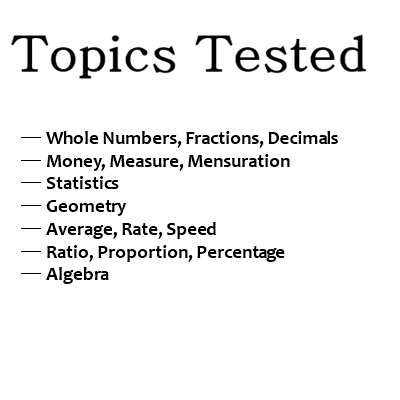Maths Topics tested

Most of the topics that are tested are built up on concepts that have been introduced since Primary 1.  From the emphasis on the  four operations ( + – X and  ÷) to   various skills and processes which are  required to solve the problem a vast array of problem solving techniques need to be implemented.

## Methods used to solve  Long Answer questions

There are several different methods which can be used to solve Long Answer questions.  The most common method of solving structured long answers is using the Model method.

This method is promoted in solving long answer questions as pupils can represent the mathematical relationships in a problem pictorially. This helps them understand the problem and plan steps for the solution. The pictorial form also helps pupils visualise what could otherwise be abstract concepts. (Fractions, Ratio & Percentage)

There are other methods which can be used to solve the Long Answer question when using the Model method doesn’t seem relevant to the posed question.

The other methods that are used are

– List and Check

– Looking for a pattern

– Working backwards.

I personally think it is important for the student to know how to use the various different methods that they have been taught in school.

In the next post I will be sharing with you how I  help Monkey boy reinforce the concepts he has learned in Maths.Post in this series

9) Bridging the Gap- Upper and Lower Primary Science

10) Bridging the Gap-  Moving from Lower to Upper Primary

11) Bridging the Gap- Moving from Lower to Upper Primary ( Part 2)